Site Map

## Sudoku: step-by-step solution of the puzzle below# Solving a Sudoku Puzzle

### The Goal of the Puzzle, and the Notation Used Here

This is a step by step solution of a random Sudoku puzzle, intended to demonstrate the method of solution. Follow the steps and you will get a good idea of how to do it. The array used is pictured above; it is suggested you bring it up on the screen (in larger format) by clicking here, print it and then use a pencil to fill in the empty squares, following the steps outlined below. Some intermediate steps will be shown, but not all.

Sudoku is a puzzle from Japan, based on what mathematicians call "Latin Squares"--square arrays of numbers in which every number appears exactly once in any row and in any column (different letters, colors or symbols may be used in place of the numbers).

A Sudoku puzzle contains 9 rows of 9 numbers, each containing (1, 2, 3 ... 9) in a different order, and the same holds for the columns. In addition, however, each Sudoku array can be divided into 9 smaller 3-by-3 squares, each of which which also must contain all the numbers (1,2,3....9). In fact many such arrays exist.

The challenge of the puzzle is--given just some of the numbers of a specific array, fill in the missing ones, using only logical deduction based on the above requirements.

The puzzle consists of a square array of 81 cells, which can be divided into 9 columns, (counted from the left), each containing 9 cells. In each column, cells are numbered from the bottom.

Or else, it can be divided into 9 rows (counted from the bottom), each containing 9 cells. In each row, cells are numbered from the left.

A notation like 6 (3,2) will mean the number "6" in cell 2 of column 3

Extra division lines (or darker ones) divide the array into 9 boxes, each with 3 rows of 3 cells each. To define the positions of boxes, it is easiest to classify them by stacks and tiers:

Three boxes in a vertical columns will be called a stack
(distinguishing left, right or middle stacks)
Three boxes in a horizontal row will be called a tier
(distinguishing bottom, top or middle tiers)

The requirement of the puzzle:
Every column, row and box must contain all the nine numbers 1,2,3 ... 9.
No number may be repeated, none can be left out.

### Filling the Missing Numbers

Numbers entered as part of the solution will be introduced in the order in which they are derived, numbered in parentheses6 (3,2)
Each of the 3 boxes in the first stack on the left must contain a "6", each in a different column. The top and middle boxes have it in columns 1 and 2, therefore the bottom box) must have it in column 3. Only one choice exists there.
Solution rule SR-1: If 3 boxes contain 3 parallel columns, the same number must appear in each column in a different box.
Similarly, If 3 boxes contain 3 parallel rows, the same number must appear in each row in a different box.

     4 (5,2)
Arguing as in , there must be a "4" in the middle column of the bottom box in the middle stack. Two empty cells exist in the column, but the top one is disqualified, because the same row already contains a "4" and the same number cannot appear in it twice.
Therefore, only one choice remains.

Hint:
in using rule SR-1 for a column, look for rows which disqualify some cells.
in using rule SR-1 for a row, look for columns which disqualify some cells.

     4 (8,1)
Each of the 3 rows of the bottom boxes needs a "4" , each in a different box. Following the above example, we expect a "4" in one of the bottom cells of the middle box on the bottom. Two empty cells exist there, but the one in the last column is disqualified, because there already is a "4" in it. There is only one choice remaining (OOCR for short).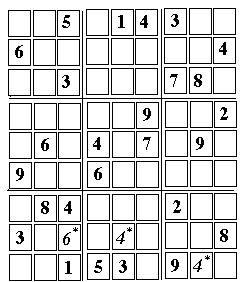9 (2,2)
There must be a "9" somewhere in bottom left box. It cannot be in its bottom row (which already has a 9), nor in the left column (same reason). OOCR--the middle cell of the box.
Note that only step  allowed this deduction. Thus  naturally follows  as we go from the easy part to the more difficult. Try to find the "natural order" of steps!

     5 (1, 3)
There must exist a "5" in the same bottom left box. It cannot be in the 3rd column (which has a "5" already), nor in the bottom row (same reason). There is OOCR.
Note that again, only steps  and  have made this possible. Often after you have filled a cell (as in step , it is a good idea to look around (in many cases nearby--but sometimes not) and see if that step has helped fill another cell.

     9 (3, 8)
Applying SR-1 (see ) to the 3 appearances of "9" in the 3 columns furthest to the left. The top box in the first stack (left top) needs a "9," and we have OOCR.
Note: this could already have been deduced as step 

     8 (1, 9)
The same top left box needs an "8." It cannot be in column 3 (it is fall filled in that box), nor in column 2 (it already has an 8). Column 1 has two empty cells, but the lower one is disqualified by the "8" in its row. The top left corner is the OOCR.
     8 (6, 1)
Each of the bottom 3 rows must have an "8", each in a different box. Thus the middle box needs put it in the lowest row, which has OOCR.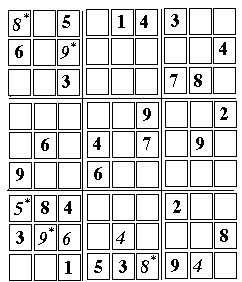Note: this step could have been the very first one, too, or undertaken any time until now.

     6 (9,1)
This is an example of using an entry without knowing its exact place.
In the bottom tier, the number "6" should appear once in every box. In the middle box there, it cannot appear in the bottom row (which is already full), nor can it appear in the middle row, which is disqualified by the "6" placed to its left in step . It therefore must be somewhere in the top row, whose three cells are currently empty.

Where exactly it will appear, one cannot tell. But wherever that is, it disqualifies the top row in the third box (the box at bottom right). The middle row is already disqualified, so the "6" must be somewhere in the bottom row, which has OOCR.

     9 (9, 9)
The top corner cell seems a good target for trying out a new approach.
The same number cannot appear twice in any row, column or box. Therefore, we look for cells whose row, column and box contain between them a wide variety of numbers.

If that variety includes all numbers except for one, that remaining number is the only choice remaining. We can call this Solution Rule 2 or SR2.For the upper right corner cell--its row contains (8, 5, 1, 4, 3), its column (9, 4, 2, 6, 8) and its box (in addition to those mentioned above), (8, 7). Thus all numbers but "9" are there, and the OOCR is 9; any other number in that cell would force a duplication.

If all numbers can be accounted for, you have made a mistake somewhere.
If all but two numbers can be accounted for, the cells contains one of the missing ones. That information, unfortunately, is useful only on rare occasions.

     7 (1, 1)
The two numbers missing from the sequence 1... 9 in the left bottom box, both in the bottom row of the puzzle, are "7" and "2". But which goes into each of the two empty cells left, in the bottom left corner?

It is best to focus on the "7". It must go (among other places) into the top left box, which has 5 empty cells left. However, the bottom row in that square is disqualified by the "7" appearing elsewhere on that row, and therefore "7" must appear in one of the remaining cells, both in column 2 of the puzzle. Regardless of which of the two is occupied by the "7", it disqualifies the (2, 1) cell from holding a "7" , so that (1,1) is the OOCR.      2 (2, 1)
follows immediately from the above
     4 (1, 6)
This has a situation similar to , two cells whose contants we can derive, but not the order in which they appear. In , it was possible to derive the order. Here the order remains unknown, but still we can use the information, because no other number can fill those cells
Look over the first stack of boxes. It has a "5" in the bottom left and another one in the top right column. Therefore the middle box must have a "5" in its middle column.

The same argument may be made for "3", too. Therefore, the two numbers flanking the "6" in the middle box, above and below it, must be "5" and "3". We do not know in what order, but they occupy those cells and therefore nothing else can.

That leave little choice for placing a "4" in that box, it can only go into column 1 or 3. Column 3 is disqualified by the "4" in the box below, cell (1, 5) is disqualified by the "4" in the central box. Thus (1, 6) is the OOCR.

A similar situation exists whenever we have a stack (or tier) of 3 boxes, and in two of them, a pair of number appears in them in two columns (or rows). If there are two empty cells (plus one occupied) in the third column (row), the two numbers must appear in them. Sometimes we can derive them (as in ), but even if not, they deny those cells to any other numbers.4 (2,7)
What follows again illustrates a frequent occurrence--once a cell is solved, several others can often be assigned as well.
In the top tier, rows 8 and 9 already have "4" in them. Wth the "4" placed by the preceding step, (2, 7) is the OOCR.      7 (2, 9)
This could have actually been done after , by using SR2, described in .
     1 (2, 8)
Another application of SR2. In general, SR2 gets more useful as more cells are filled, as long as all numbers are well represented. Sometimes however the filled array is short of two numbers, say 2 and 8. Then, whenever we assemble enough filled cells to try SR2, always the same two numbers are missing. Such puzzles are the most difficult! Usually, however, there exists a loophole somewhere--if you can find it.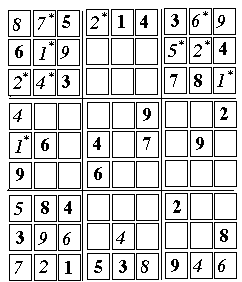Now many opportunities are opened.

     2 (1, 9)
This was the only number missing in the top left box.
     1 (1, 5)
This was the only number missing in the first column.
     2 (4, 9)
Applying SR2. From now on, gradually more and more opportunities exist for applying SR2. The trick is to find an empty cell "ripe" for such application!

     6 (8, 9)
This was the only number missing in the top row.
     1 (9, 7)
Each row in the top tier must have a "1" in a separate box. . The two top rows and the first two boxes already have theirs, and this is the only solution open.
     2 (8, 8)
Only two cells are left and one must contain "2". It cannot be in column 7, which already has a "2", so OOCR.
     5 (7, 8)
This was the only number missing in the top right box.
     4 (7,4)
This is an example of "crossover," another useful trick. The middle tier has two rows with "4" and one without. The right hand stack of boxes has two columns with "4" and one without. Where missing "4" must be on the "empty row," and similarly it also must be on the "empty column." Therefore, wherever the two cross, that is the cell it occupies.
     2 (6, 2)
A bit like another "crossover." In the bottom tier, only the middle row is empty of "2", so that number must be there. Of the intersecting columns, , two are empty, but one of them is blocked by a "4" entered in step . Thus OOCR.
     1 (7, 2)
using SR2.. (After performing SR2, always check by locating and ticking off all nine numbers in order).
     6 (7, 6)
Only two numbers ore missing in column 7, and a check shows they are 6 and 8. However, the 6 cannot go in (7, 5) because the row already contains a "6". So OOCR.
     8 (7, 5)
follows from the above.
     5 (8, 2)
Similar to : only two empty cells remain in the second row from bottom, and the missing numbers are 5 and 7. However, 5 cannot go into (4, 2) because another 5 is right below, hence OOCR.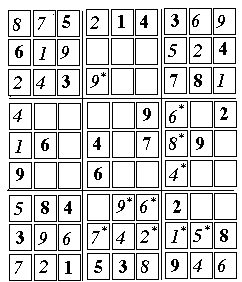7 (4, 2)
follows from the above
     9 (4, 7)
using SR2
     9 (5, 3)
using a cross-over similar to the one in .
     6 (6, 3)
Similar to  and : only two empty cells left in the middle box of the bottom tier, and the missing numbers are 1 and 6. However 6 can't go into (4, 3) which is right below another 6, so OOCR.
     1 (4, 3)
follows from the above.
     5 (6, 7)
Applying SR2.
[36-7]     1 (6, 4) ,     3 (6, 8)
Now only two empty cells remain in column 6, to be filled by 1 and 3. One of these is disqualified by a "1" in the same row, so OOCR.
     6 (5, 7)
Last remaining free cell in row 7.
      7 (5, 8)     8 (4, 8)
Only two empty cells left in row 8, for 7 and 8; however, one column already has a 7, so OOCR.2 (3, 5)
using SR2.
      3 (9, 5)     5 (5, 5)
Only two cells left empty in row 5, for placing 3 and 5. However, one of them is not suitable for 3, having a 3 in the same column, at the bottom. So OOCR.
     2 (5, 4)
derived by cross-over similar to the one in .
      3 (4, 6) , 8 (5, 6)
Only two cells left empty in row 5, for placing 3 and 8. The same 3 active in  and  again ensures that OOCR.
      3 (2, 4)     5 (2, 6)
Only now can a decision be made, which of the two cells in  holds a 3 and which holds a 5 ! The "3" placed in the preceding step determines the lower one is a 3 and the upper one is a 5: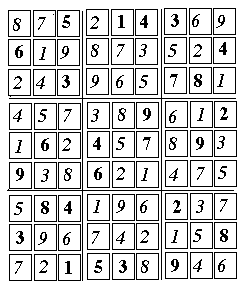The remaining few cells are so easily filled, they will not be discussed.

## Further Exploration

An article "The Science behind Sudoku" by Jean-Paul Delahaye appeared in Scientific American, June 2006, p. 80-87.